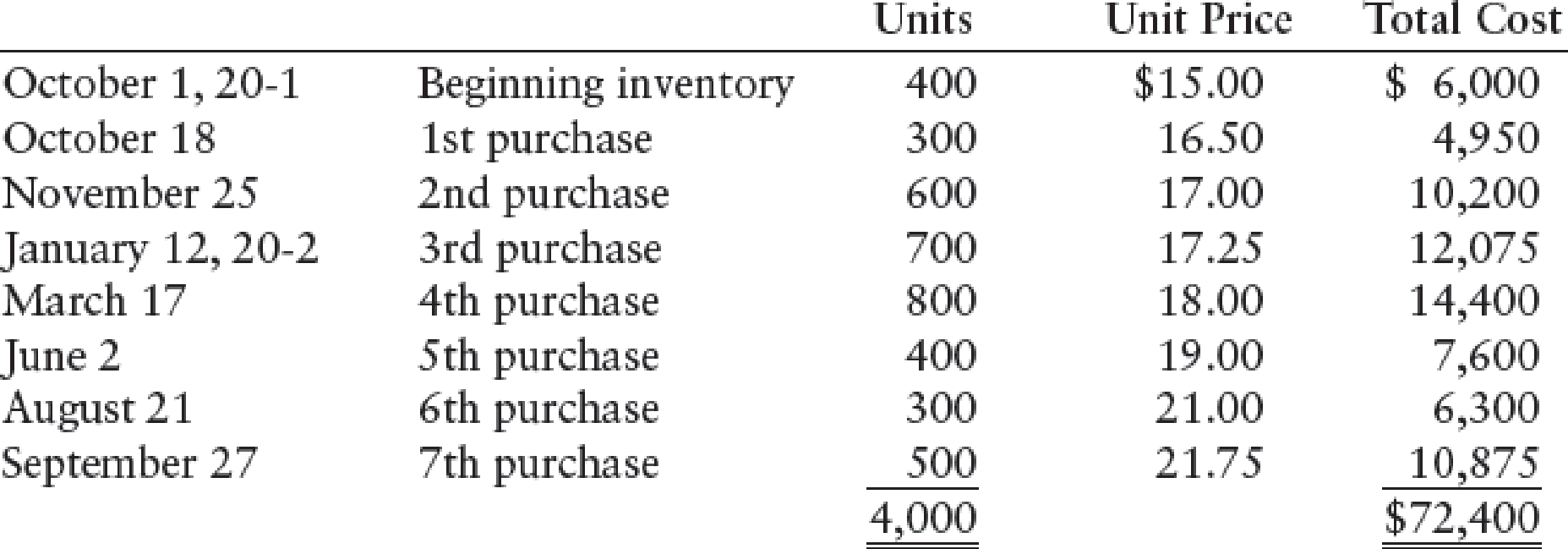Chapter 13, Problem 6SPB

Chapter
Section
Textbook Problem

SPECIFIC IDENTIFICATION, FIFO, LIFO, AND WEIGHTED-AVERAGE Boyce Company’s beginning inventory and purchases during the fiscal year ended September 30, 20-2, were as follows:Use the following information for the specific identification method.There are 900 units of inventory on hand on September 30, 20-2. Of these 900 units:REQUIREDCalculate the total amount to be assigned to the cost of goods sold for the fiscal year ended September 30, 20-2, and ending inventory on September 30, 20-2, under each of the following periodic inventory methods: 1. FIFO 2. LIFO 3. Weighted-average (round calculations to two decimal places) 4. Specific identification

1.

To determine

Calculate the total amount of cost of goods sold and cost of ending inventory on September 30, 20-2 under FIFO method (Periodic inventory system).

Explanation

Periodic Inventory System: It is a system in which the inventory is updated in the accounting records on a periodic basis such as at the end of each month, quarter or year. In other words, it is an accounting method which is used to determine the amount of inventory at the end of each accounting period.

First-in-First-Out (FIFO): In First-in-First-Out method, the first purchased items are sold first...

2.

To determine

Calculate the total amount of cost of goods sold and cost of ending inventory on September 30, 20-2 under LIFO method (Periodic inventory system).

3.

To determine

Calculate the total amount of cost of goods sold and cost of ending inventory on September 30, 20-2 under weighted average cost method (Periodic inventory system).

4.

To determine

Calculate the total amount of cost of goods sold and cost of ending inventory on September 30, 20-2 under specific identification method (Periodic inventory system).

Still sussing out bartleby?

Check out a sample textbook solution.

See a sample solution

The Solution to Your Study Problems

Bartleby provides explanations to thousands of textbook problems written by our experts, many with advanced degrees!

Get Started

Find more solutions based on key concepts